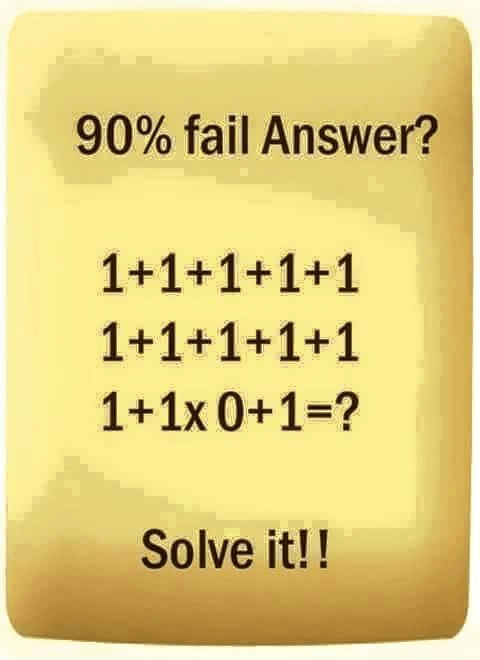Categories

# 1 + 1 + 1 + 1 + 1 90% Fail1 + 1 + 1 +1 + 1
1 + 1 + 1 + 1 + 1
1 + 1 x 0 + 1 = ?

Most people won’t agree with this answer. Answer they come up with is 30, as they solve this as below:

1 + 1 + 1 + 1 + 11 + 1 + 1 + 1 + 11 + 1 x 0 + 1
i.e. 4 + 11 + 3 + 11 + 0 + 1 = 30

The first two lines don’t have all the necessary symbols to express a problem to be solved, nor are you allowed in mathematical typography to do a line break between two digits in a number. In other words, you can’t combine the last 1 in the first row with the first 1 in the second row and assume you’re reading an 11. That’s not allowed in typography. So the first two lines have no relationship to each other or the third and can be ignored as mathematical garbage. The third line, however, does express a complete problem to be solved, with all the necessary symbols.

Now, all you need to solve is 1 + 1 x 0 + 1 = ?

BIMDAS tells you how to solve this: Brackets, Indices, Multiplication, Division, Addition, Subtraction are the order of the operations you solve. So, the problem actually looks like this: 1 + (1 x 0) + 1 = 1 + 0 + 1 = 2.